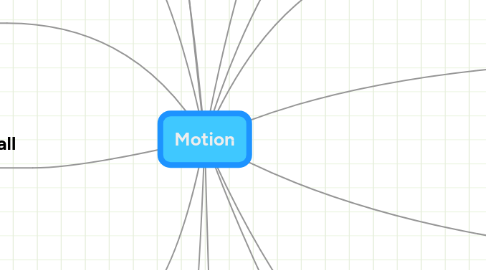# Motion

Get Started. It's FreeMotion## 1. Acceleration

### 1.2. indicates any change in the velocity vector

1.2.1. change in direction

1.2.2. increase in speed

1.2.3. decrease in speed

1.2.4. simultaneous change in speed and direction

### 1.3. To find it:

1.3.1. the change in velocity divided by the corresponding time interval

1.3.2. meters per second squared

1.4.1. net force and velocity point in opposite directions=slowing down

1.4.2. Net force and velocity point in same direction= speeding up

1.4.3. Straight-line motion= acceleration is wither same direction as velocity or in the opposite direction to the velocity

2.3.1. Direction

2.3.2. Distance

## 4. Velocity

### 4.2. Average velocity

4.2.1. does not reveal information about the motion during the time interval

4.2.2. product of the vector, the displacement, and a positive scalar, the inverse of the time interval

4.2.3. x-axis

4.2.3.1. represented on the graph as x(t)

### 4.3. Instantaneous velocity

4.3.1. a vector

4.3.2. magnitude is the speed

4.3.3. direction of motion

4.3.4. used to calculate the displacement of the object during a very short interval

4.4.1. sloping portions on graphs indicate=movement

4.4.2. a horizontal position=position has not changed

4.4.3. steeper the graph=faster speed

4.4.4. sign of the slope indicates direction of motion

4.4.4.1. positive slope

4.4.4.2. positive slope=motion in the +x-direction

4.4.4.3. negative slope=motion in the -x-direction

## 5. Newton's second law

### 5.3. Applying to problems

5.3.1. Decide what objects will have Newton's second law applied to them

5.3.2. Identify all the external forces acting on that object

5.3.3. Draw a FBD to show all the forces acting on the object

5.3.4. Choose a coordinate system

5.3.5. find the net force by adding the forces as vectors

5.3.6. Relate the net force to acceleration

5.3.7. Relate the acceleration to the change in the velocity vector during a time interval of interest

## 6. Constant net force

### 6.1. equations

6.1.1. change of velocity is equal to the final velocity minus the initial velocity = acceleration times the change in time

6.1.2. refer to page 108 and 109

## 7. Visualization

### 7.1. Motion diagrams

7.1.1. position

7.1.2. velocity

7.1.3. Acceleration

8.2.1. neglible

## 9. Motion of projectiles

### 9.2. Projectiles

9.2.1. objects in free fall

9.2.2. motion takes place in vertical plane

### 9.3. Angle of elevation

9.3.1. angle of initial velocity above the horizontal

### 9.4. Trajectory

9.4.1. path it takes in air

## 10. Apparent Weight

### 10.2. elevator example

10.2.1. upwards the weight will be great than the true weight

10.2.2. downwards the weight will be less than the true weight

## 11. Air resistance

### 11.1. Drag force

11.1.1. increases as speed increases

11.1.2. the larger the surface area the more air is needed to be pushed out of the way

### 11.2. Terminal velocity

11.2.1. the acceleration is zero

### 11.3. Terminal speed

11.3.1. the magnitude of the terminal velocity is:

11.3.2. depends on:

11.3.2.1. size

11.3.2.2. shape

11.3.2.3. mass

## 12. Vectors

### 12.2. Problem solving

12.2.1. Vectors in different direction, Subtraction: is to add its opposite

12.2.1.1. has same magnitude but opposite direction

12.2.2. Multiplication

12.2.2.1. multiplyig a vector by the scalar -1 reverses the vector's direction while magnitude is unaffected

12.2.3. Subtraction : used to find displacement

## 13. Words of the Day

### 13.1. Forestall

13.1.1. to predict what is ahead

### 13.2. Modus operandi

13.2.1. "mode of operation"

### 13.3. Ne Plus ultra

13.3.1. the most profound degree

### 13.4. Ithaca

13.4.1. the journey

13.5.1. cures

### 13.6. Explore

13.6.1. To investigate systematically; examine

### 13.7. Red Herring

13.7.1. something intended to divert attention from the real problem or matter at hand

### 13.8. Mash-Up

13.8.1. combining data from more than one source into a single integrated tool

### 14.1. Interval

14.1.1. The amount of time between two specified instants, events, or states

14.2.1. rise/run

### 14.3. Mass

14.3.1. measure of its inertia

### 14.4. Weight

14.4.1. is the magnitude of the gravitational force acting on it